# Coming Soon: Capability to Constrain Multiphase Equilibrium CalculationsDates:

The next release of Process Safety Office® SuperChems™ will provide the capability to constrain multiphase chemical and physical equilibrium calculations. This is an important upgrade for all SuperChems™ models using Gibbs free energy minimization including streams, and the Gibbs free energy model. The release will also help users better develop global kinetics (rates and stoichiometries) from calorimetry data where constraints are measured.

## Can Specify User-Defined Constraints

When users create a new mixture or when users edit the number of compounds in a mixture, they can specify user-defined constraints, including both equality and inequality constraints.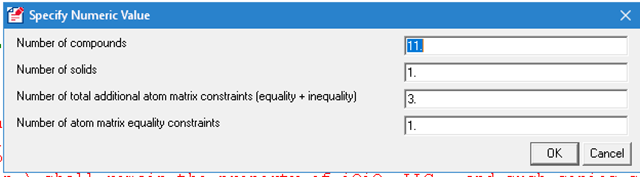## Can Constrain Based On Actual Measurements

Equilibrium states are usually difficult to achieve in liquid and heterogeneous reactions. In the next release, users can constrain the equilibrium calculations based on actual measurements, such as the final ratio of non-condensable gas, to force the equilibrium calculation to match reaction data for rate-limited reactions. Users can also use constraints when calculating VLLE to restrict a specific chemical species to one or more phases. A user specifies the constraints by directly editing the mixture after setting the number of constraints. The figure below shows a mixture that is used to model the combustion of methane. Note that it has three user-defined constraints: one equality constraint and two inequality constraints.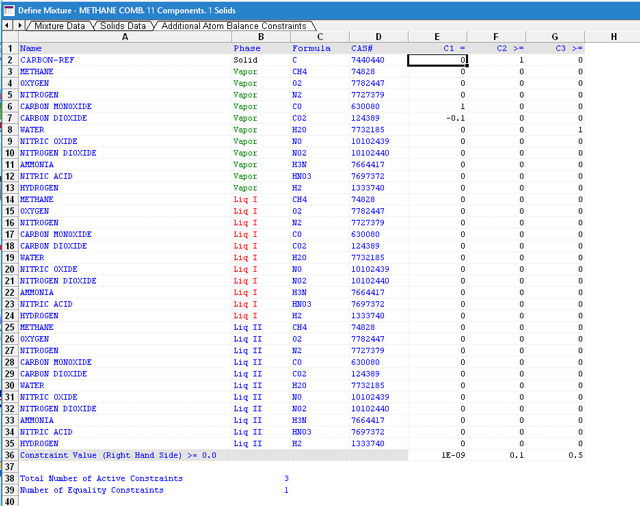The equality constraint constrains the molar ratio of carbon monoxide to carbon dioxide to 10 % or 0.1 for the vapor phase only.

nCO/nCO2 = 0.1 or nCO – 0.1 nCO2 = 0.0

1 and -0.1 are shown for the equality constraint and the right hand side is set to 1.0E-9 to prevent nCO from going to 0. These types of constraints are preferred to absolute constraints since the total number of moles can change depending on the initial number of moles/flow rate and chemical conversion.

The second constraint is an inequality constraint. It constrains the final number of carbon to 0.1 mole or greater. This is an absolute constraint and regardless of the starting number of moles, it will require that the equilibrium number of moles of carbon cannot be less than 0.1 mole.

nC >= 0.1

The third constraint is also an absolute non-equality constraint which constrains the final number of water moles to 0.5 or greater.

nH2O >= 0.5

The figure below shows that when the Gibbs free energy code is run, starting with two moles of fuel and oxidant, the following solution is returned. Note that the final number of carbon moles is 0.1 and the final number of water moles is 0.666 where both inequality constraints are satisfied. Also note the ratio of CO/CO2 is 0.1 which satisfies the equality constraint.

This solution is a constrained equilibrium end state yielding a total energy release of -969.43 cal/g.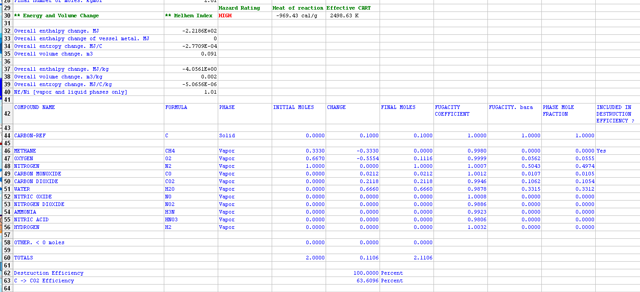Were the user to run the calculation without the additional constraints, the figure below shows the following equilibrium estimate for the final state.Note the total energy release is -1167.59 cal/g and the stoichiometry is different, as the number of C moles and CO moles is zero.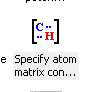SuperChems™ provides a simple additional utility that can populate the constraints from some typical situations.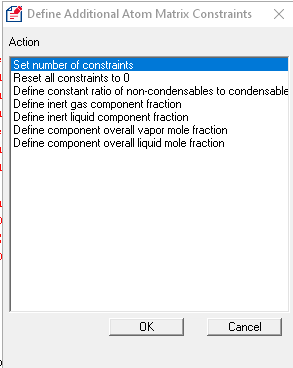These new features in SuperChems™ should prove very useful when dealing with multiphase reactions or VLLE systems. Please note that absolute constraints should be avoided unless the user is clearly aware of the starting number of moles and how that compares to the absolute constraint value(s). It is preferred to create constraints that are relative to either the total number of moles in one or more phases, or a specific component, etc.

## Don't Have SuperChems™?

This versatile software tool provides an integrated platform for pressure relief and flare systems (PRFS) evaluation and design, consequence analysis (emission/flow, fire, dispersion, and explosion dynamics), quantitative risk assessment (QRA), transportation risk assessment (TRA), and chemical reactions modeling. SuperChems™ overcomes the shortcomings of alternative simple design and evaluation methods leading to over-design, or worse yet, under-design.